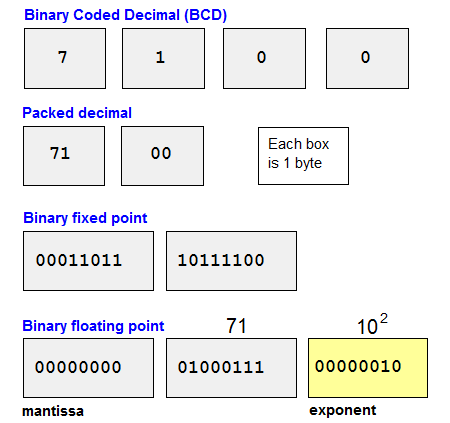Definition: binary numbers

In the computer, all data are represented as binary digits (bits), and eight binary digits make up one byte. For example, the upper case letter A is 0101001. Numbers however can take several forms. They can retain their decimal identity or they can be in pure binary form. See binary and ASCII chart.

Binary Coded Decimal (BCD)

BCD encodes each decimal digit in a single byte. For example, the number 7100 would take four bytes. A variation, called "packed decimal," encodes two decimal digits in one byte, and the number 7100 would take only two bytes.Binary Fixed Point
This method converts the entire decimal number into a binary number. The number 7100 would require at least two bytes as in the example above. Binary numbers are calculated faster than decimal (BCD) numbers. See binary values.

Binary Floating Point
Floating point allows very small fractions and very large numbers to be maintained and calculated quickly. Both the mantissa (significant digits) and the exponent (power to which the base is raised) are in binary. In this above example, the mantissa is 71, and the exponent is 2. See floating point.

Bytes  Bits   Values
1      8      0 to 255
2      16     0 to 65,535
4      32     0 to 4,294,967,295